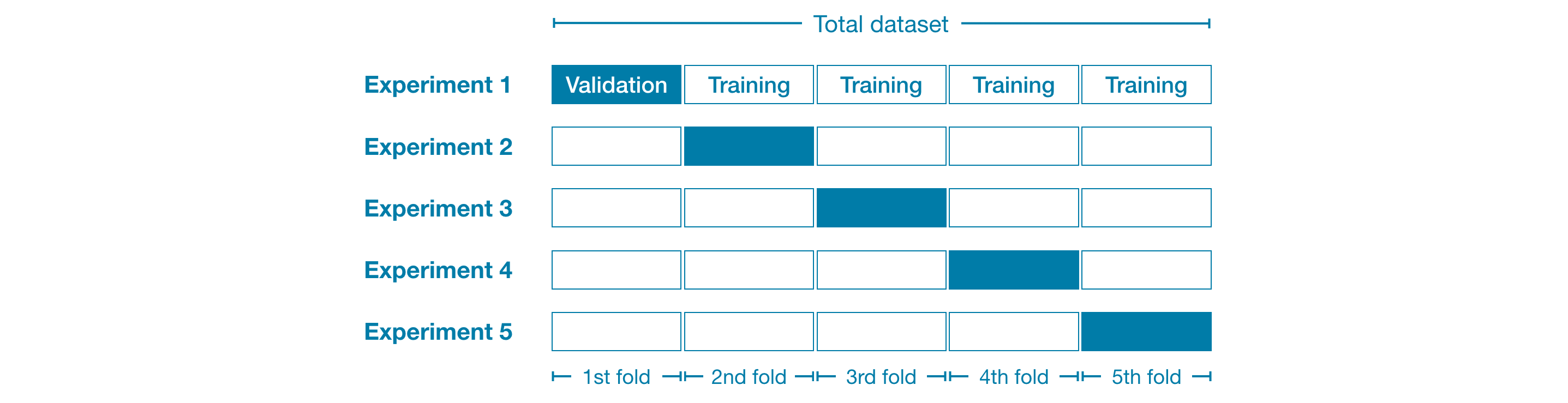# 机器学习- Sklearn (交叉验证和Pipeline)

2019/04/10 10:10

• Cross_validationfrom sklearn.model_selection import cross_val_score
scores = cross_val_score(estimator=my_pipeline, X=X, y=y, cv=5,scoring = "neg_mean_absolute_error")

• Pipeline

咱们前面花了很多的时间讲了feature engineering， 模型训练等等知识，咱们可以看见data preprocess的过程还是很复杂的，例如有missing value handling， categorical data encoding等等这些步骤，然而，每个项目中的数据都是不一样的，这就让咱们的数据进行preprocess的时候会非常复杂，没换一个dataset，咱们就得重新来一遍，非常不方便，这时候聪明的人类就想到了，能不能通过定义一部分参数，来实现把这些繁杂的过程格式化呢？？？这时候pipeline就出来了。咱们现在先把代码贴出来，然后在一条一条的解释一下pipeline的步骤

#Step1: data preparation
import pandas as pd
from sklearn.model_selection import train_test_split
X = melb_house_price.drop(["Price"],axis = 1)
y = melb_house_price.Price
X_train_full, X_val_full,y_train,y_val = train_test_split(X,y,train_size=0.8,test_size=0.2,random_state=1)

numerical_cols = [col for col in X_train_full.columns if X_train_full[col].dtype in ['int64','float64']]
categorical_cols = [col for col in X_train_full.columns if X_train_full[col].dtype == 'object' and X_train_full[col].nunique()<10]
my_cols = numerical_cols+categorical_cols

X_train = X_train_full[my_cols].copy()
X_val = X_val_full[my_cols].copy()

#Step2: define preprocess steps
from sklearn.impute import SimpleImputer
from sklearn.pipeline import Pipeline
from sklearn.preprocessing import OneHotEncoder
from sklearn.compose import ColumnTransformer
from sklearn.metrics import mean_absolute_error
#2.1 define transformer of numerical data
numerical_transformer = SimpleImputer(strategy="mean") #missing value handling
#2.2 define transformer of categorical data #(Firstly handle the missing value and then secondly encoding the )
categorical_transformer = Pipeline(steps=[
("Imputer",SimpleImputer(strategy="most_frequent")),
("Onehot",OneHotEncoder(handle_unknown='ignore'))]
)
#2.3 data preprocessor
preprocessor = ColumnTransformer(
transformers = [
("num", numerical_transformer, numerical_cols),
("cat",categorical_transformer,categorical_cols)]
)
#step2.4: model selection and definition
from sklearn.ensemble import RandomForestRegressor
model = RandomForestRegressor(n_estimators = 100, random_state = 0)
#step2.5: bundle preprocessor and model
my_pipeline = Pipeline(
steps = [
('preprocessor', preprocessor),
('model',model)]
)

#step3: fitting the model
my_pipeline.fit(X_train,y_train)

#step4: predicts
predictions = my_pipeline.predict(X_val)

#step5: evaluate the modle
print(mean_absolute_error(y_val,predictions))

• 总结

上面已经展示的交叉验证cross_validation和pipeline的两个知识点的一些基本原理和应用。在这里cross_validation的形式和原理是重点，已经cross_validation的作用和原因要理解，至于应用方面就很简单了，重点关注一下里面的一些参数设置就行了。pipeline能够大大的减少咱们数据处理方面的工作量，相当于把数据的preprocessor打包了，并且结构化，具有很高的复用性，这里重点需要了解pipeline的应用的流程以及为什么是这个流程，然后是每个流程的作用。上面就是这一节补充的2个sklearn应用中的小知识点。

0
0 收藏

### 作者的其它热门文章0 评论
0 收藏
0